Ex 4.1

Chapter 4 Class 7 Simple Equations
Serial order wise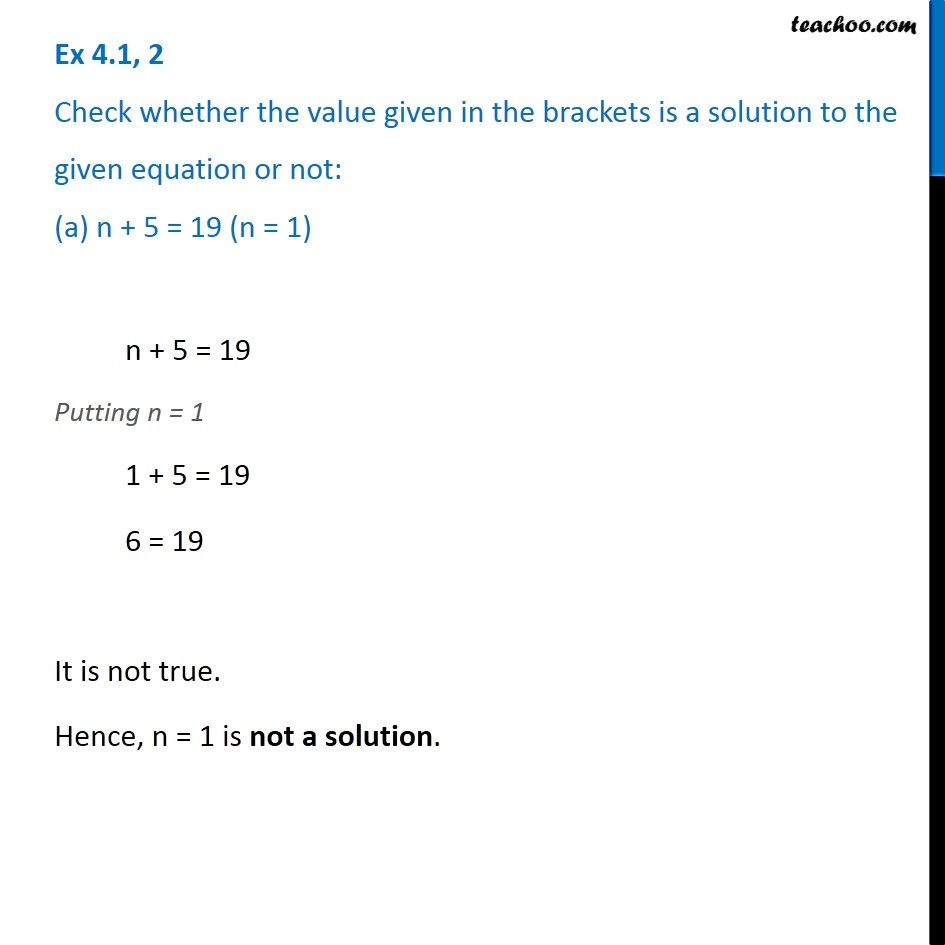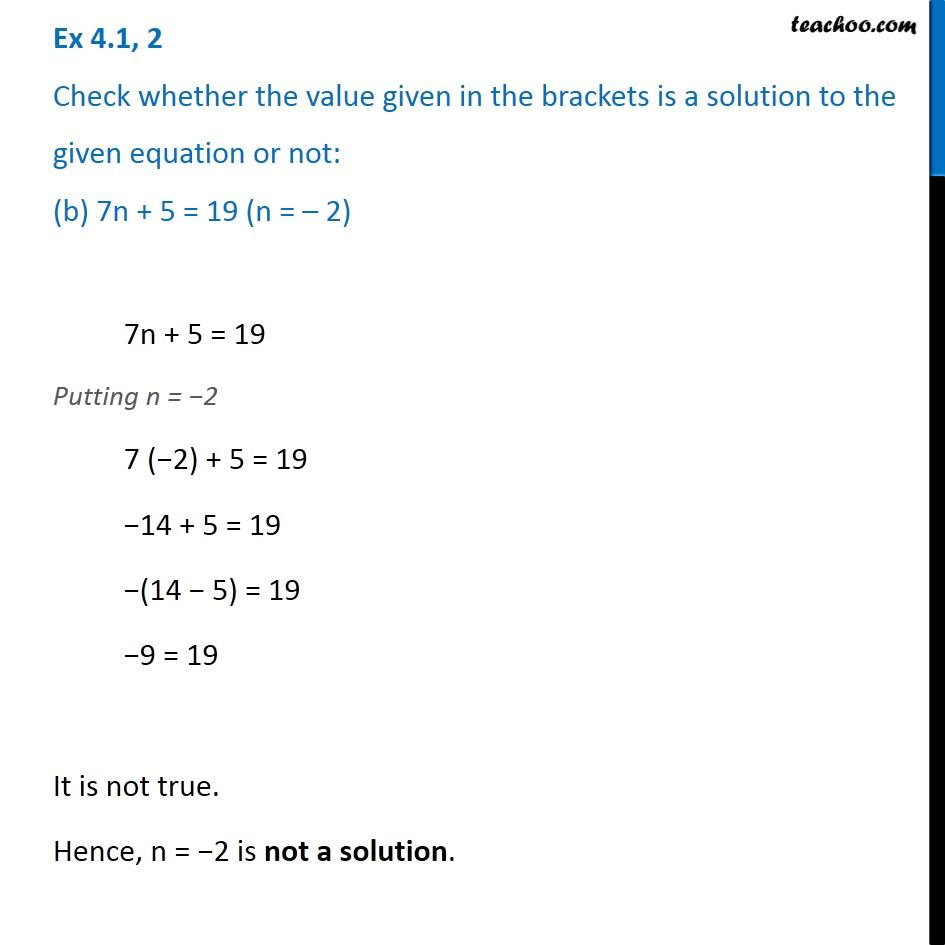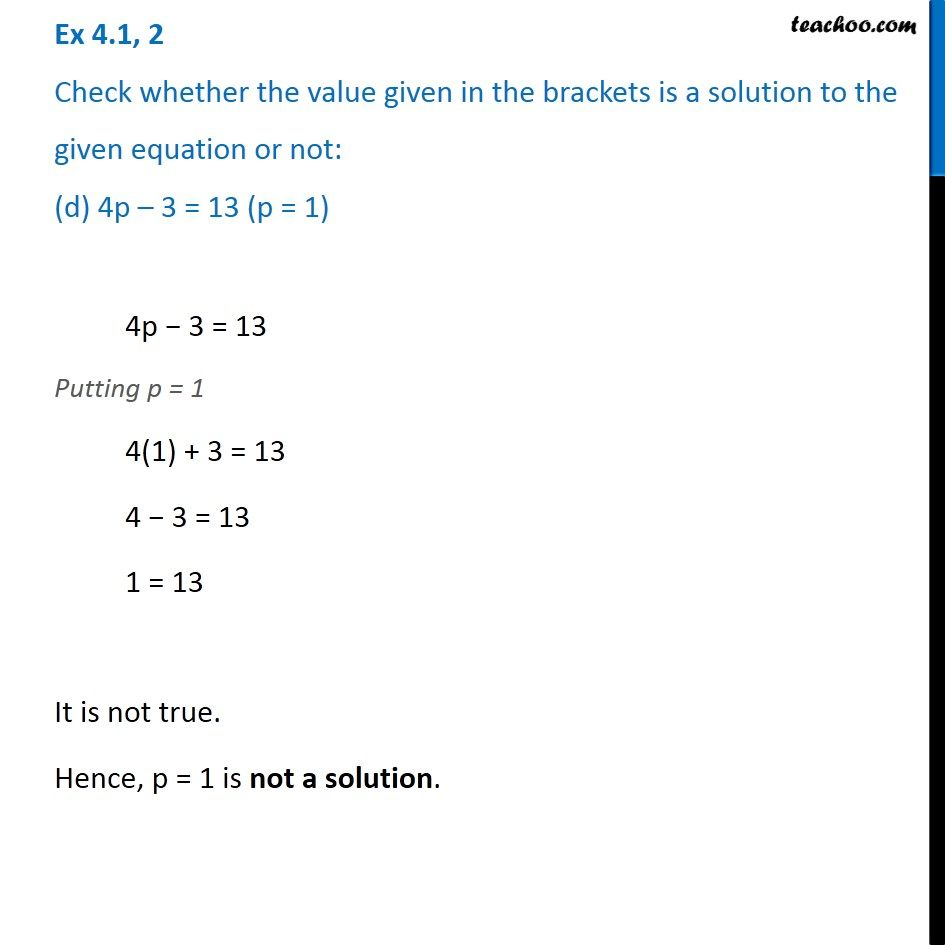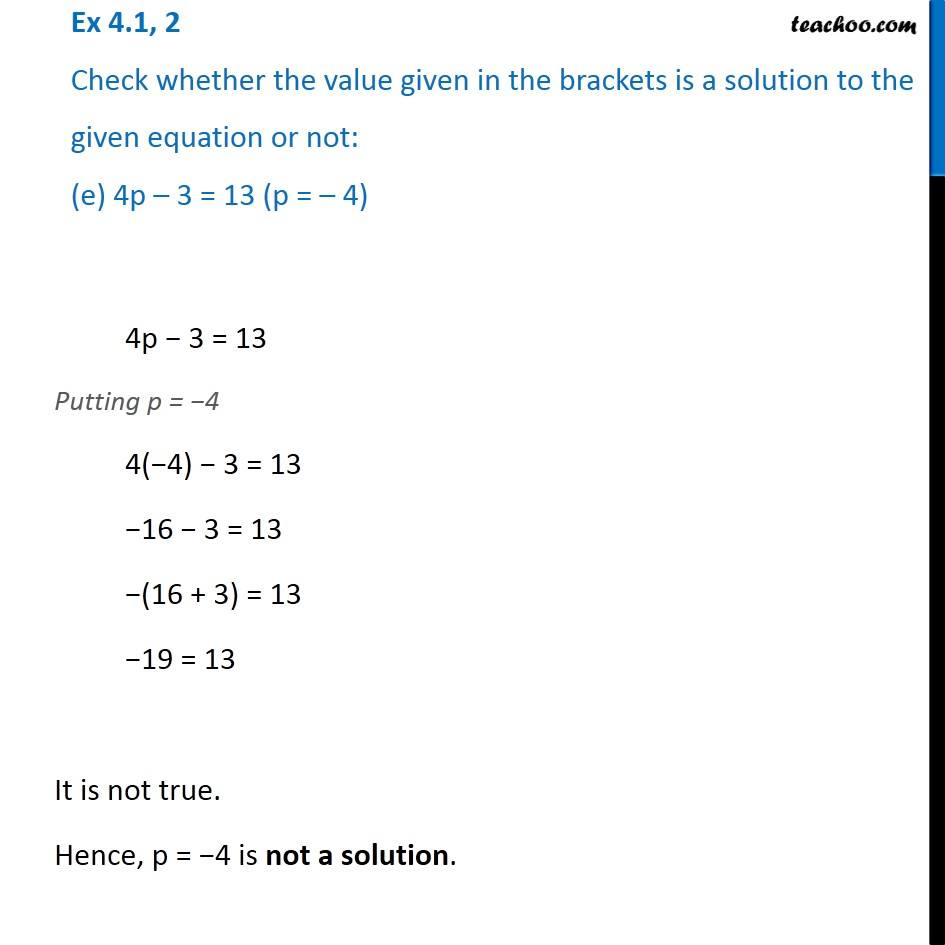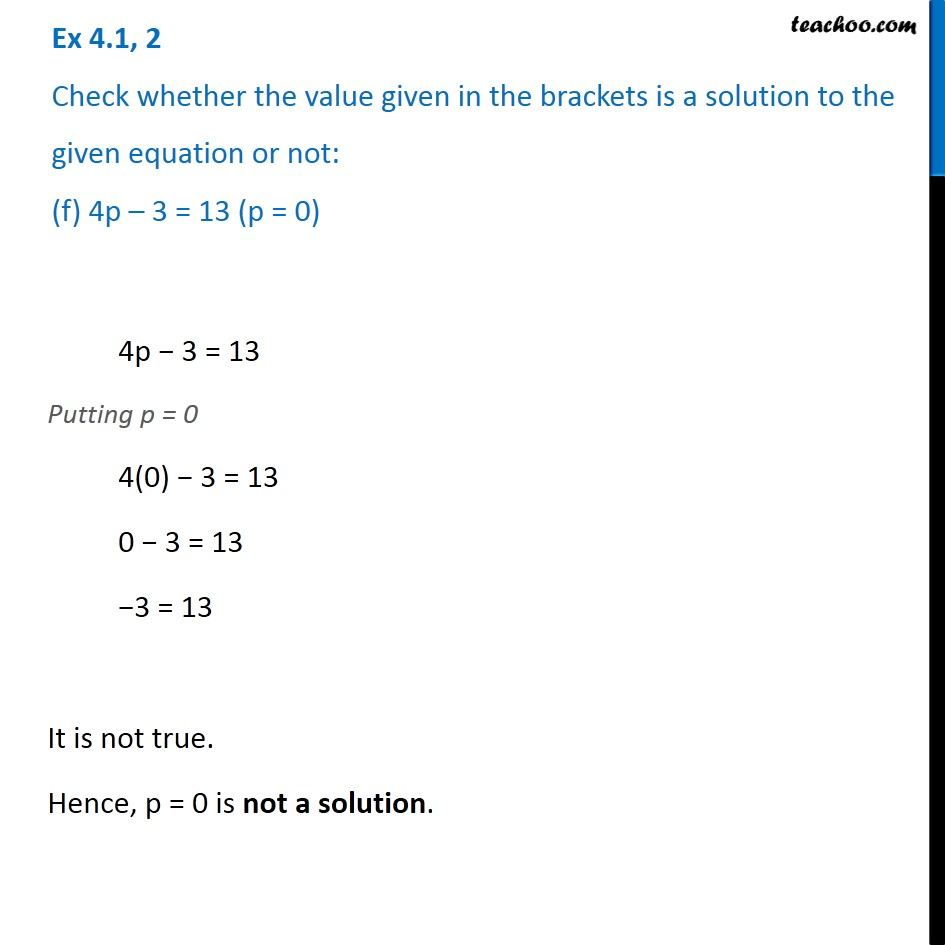Learn in your speed, with individual attention - Teachoo Maths 1-on-1 Class

### Transcript

Ex 4.1, 2 Check whether the value given in the brackets is a solution to the given equation or not: (a) n + 5 = 19 (n = 1) n + 5 = 19 Putting n = 1 1 + 5 = 19 6 = 19 It is not true. Hence, n = 1 is not a solution. Ex 4.1, 2 Check whether the value given in the brackets is a solution to the given equation or not: (b) 7n + 5 = 19 (n = – 2) 7n + 5 = 19 Putting n = −2 7 (−2) + 5 = 19 −14 + 5 = 19 −(14 − 5) = 19 −9 = 19 It is not true. Hence, n = −2 is not a solution. Ex 4.1, 2 Check whether the value given in the brackets is a solution to the given equation or not: (c) 7n + 5 = 19 (n = 2) 7n + 5 = 19 Putting n = 2 7 (2) + 5 = 19 14 + 5 = 19 19 = 19 It is true. Hence, n = 2 is a solution. Ex 4.1, 2 Check whether the value given in the brackets is a solution to the given equation or not: (d) 4p – 3 = 13 (p = 1) 4p − 3 = 13 Putting p = 1 4(1) + 3 = 13 4 − 3 = 13 1 = 13 It is not true. Hence, p = 1 is not a solution. Ex 4.1, 2 Check whether the value given in the brackets is a solution to the given equation or not: (e) 4p – 3 = 13 (p = – 4) 4p − 3 = 13 Putting p = −4 4(−4) − 3 = 13 −16 − 3 = 13 −(16 + 3) = 13 −19 = 13 It is not true. Hence, p = −4 is not a solution. Ex 4.1, 2 Check whether the value given in the brackets is a solution to the given equation or not: (f) 4p – 3 = 13 (p = 0) 4p − 3 = 13 Putting p = 0 4(0) − 3 = 13 0 − 3 = 13 −3 = 13 It is not true. Hence, p = 0 is not a solution.